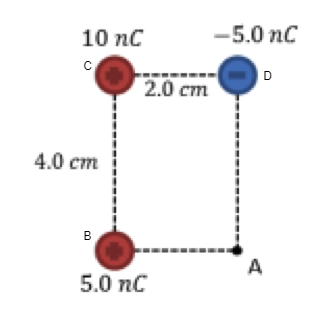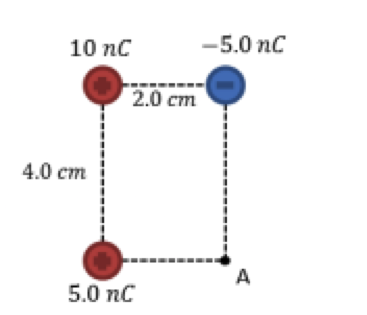# Problem: a. What is the electric potential at point A in the figure above?b. What is the potential energy of a proton at point A?

###### FREE Expert Solution

The potential at point Ais the sum of potentials due to each charge.

Given that there are many charge sources, the electric potential at a point is the sum of the potentials due to each charge.

$\overline{){\mathbf{V}}{\mathbf{=}}\mathbf{\sum }_{\mathbf{i}}{\mathbf{K}}\frac{{\mathbf{q}}_{\mathbf{i}}}{{\mathbf{r}}_{\mathbf{i}}}}$

Electric potential energy:

$\overline{){\mathbf{U}}{\mathbf{=}}{\mathbf{qV}}}$

Let's consider the labeled diagram below:a.

The potential at point A due to the charge is:

V = kqB/rAB + kqc/rAC + kqD/rAD

83% (362 ratings)###### Problem Detailsa. What is the electric potential at point A in the figure above?

b. What is the potential energy of a proton at point A?